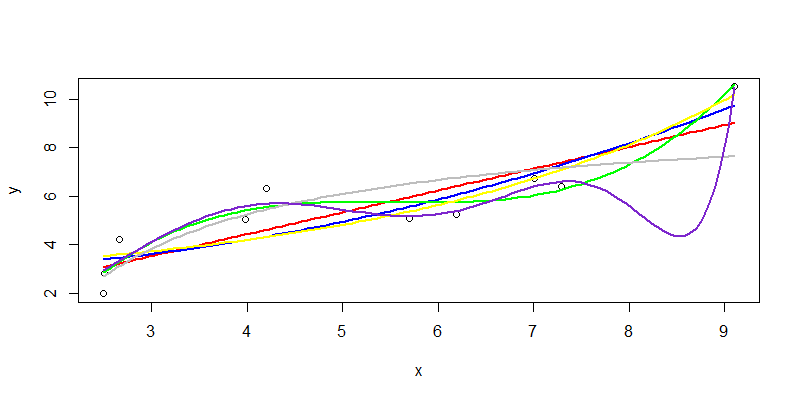• Posts tagged "线性"

# Blog Archives

## 线性和非线性的最小二乘回归

• 张丹(Conan), 程序员/Quant: Java,R,Nodejs
• blog: http://blog.fens.me
• email: bsspirit@gmail.com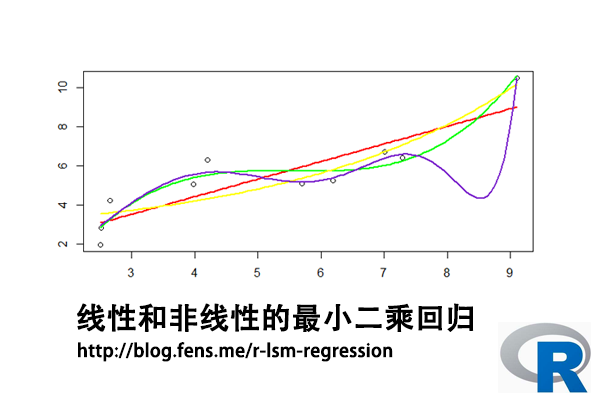1. 最小二乘法介绍
2. 最小二乘求解
3. 线性最小二乘回归
4. 非线性最小二乘回归

## 1. 最小二乘法介绍

• H, 目标值，观测值与理论值之差的平方和
• H’, 最小化的H值
• y, 观测值
• yi, 理论值
• argmin, 最小化函数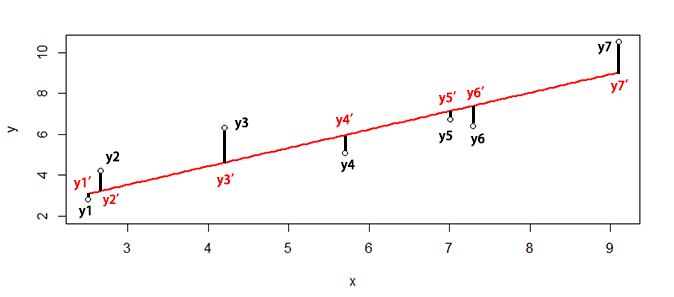``SSE=sum((y1-y1')^2+(y2-y2')^2+(y3-y3')^2+(y4-y4')^2+(y5-y5')^2+(y6-y6')^2+(y7-y7')^2)``

## 2. 最小二乘求解

``````> x<-c(6.19,2.51,7.29,7.01,5.7,2.66,3.98,2.5,9.1,4.2)
> y<-c(5.25,2.83,6.41,6.71,5.1,4.23,5.05,1.98,10.5,6.3)``````

``> plot(x,y)``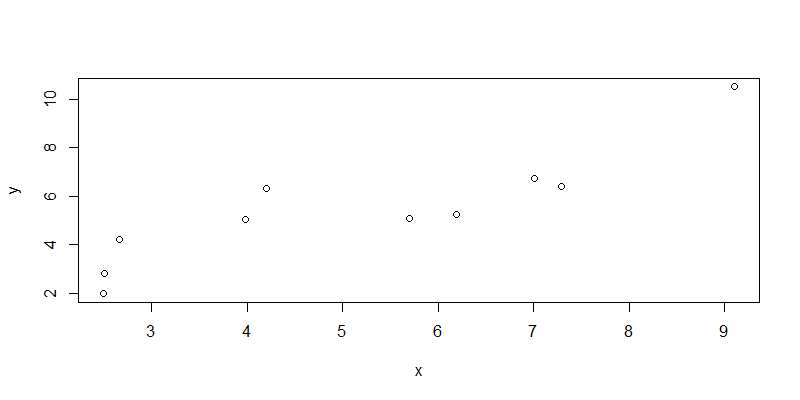``````
> fit<-lsfit(x,y);fit
\$coefficients
Intercept         X
0.8310557 0.9004584

\$residuals
 -1.1548933 -0.2612063 -0.9853975 -0.4332692 -0.8636686  1.0037250  0.6351198 -1.1022017
  1.4747728  1.6870190

\$intercept
 TRUE

\$qr
\$qt
 -17.1901414   6.2044421  -0.7047339  -0.1530724  -0.5856560   1.2766692   0.9102648
  -0.8295242   1.7584540   1.9625308

\$qr
Intercept           X
[1,] -3.1622777 -16.1718880
[2,]  0.3162278   6.8903149
[3,]  0.3162278  -0.2782874
[4,]  0.3162278  -0.2376506
[5,]  0.3162278  -0.0475287
[6,]  0.3162278   0.3936703
[7,]  0.3162278   0.2020970
[8,]  0.3162278   0.4168913
[9,]  0.3162278  -0.5409749
[10,]  0.3162278   0.1701682

\$qraux
 1.316228 1.415440

\$rank
 2

\$pivot
 1 2

\$tol
 1e-07

attr(,"class")
 "qr"
``````

• coefficients, 系数的最小二乘估计，包括截距Intercept和自变量X
• residuals, 残差
• intercept, 有截距
• qt, 矩阵QR分解。QR分解法是将矩阵分解成一个正规正交矩阵与上三角形矩阵。关于矩阵的详细操作，请参考文章R语言中的矩阵计算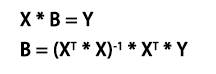• X，为自变量矩阵
• Y，为因变量矩阵
• B，为参数，需要求解
• XT，X的转置矩阵
• *, 矩阵乘法
• -1, 计算逆矩阵

``````
# 因变量矩阵
> y1<-matrix(y,ncol=1)

# 自变量矩阵，增加截距列
> x1<-matrix(c(x,rep(1,length(x))),ncol=2)

# 求解最小二乘解
> solve(t(x1)%*%x1) %*% t(x1) %*% y1
[,1]
[1,] 0.9004584
[2,] 0.8310557
``````

## 3. 线性最小二乘回归

``````
# 建立回归模型
> line<-lm(y~x)

# 查看回归模型
> summary(line)
Call:
lm(formula = y ~ x)

Residuals:
Min      1Q  Median      3Q     Max
-1.1549 -0.9550 -0.3472  0.9116  1.6870

Coefficients:
Estimate Std. Error t value Pr(>|t|)
(Intercept)   0.8311     0.9440   0.880 0.404338
x             0.9005     0.1698   5.302 0.000726 ***
---
Signif. codes:  0 ‘***’ 0.001 ‘**’ 0.01 ‘*’ 0.05 ‘.’ 0.1 ‘ ’ 1

Residual standard error: 1.17 on 8 degrees of freedom
Multiple R-squared:  0.7785,	Adjusted R-squared:  0.7508
F-statistic: 28.12 on 1 and 8 DF,  p-value: 0.0007263

# 残差平方和
> sum(line\$residuals^2)
 10.95334
``````

``````
# 设置x的取值区间
> new <- data.frame(x = seq(min(x),max(x),len = 100))

# 预测结果
> pred<-predict(line,newdata=new)
``````

``````
> plot(x,y)
> lines(new\$x,pred,col="red",lwd=2)
``````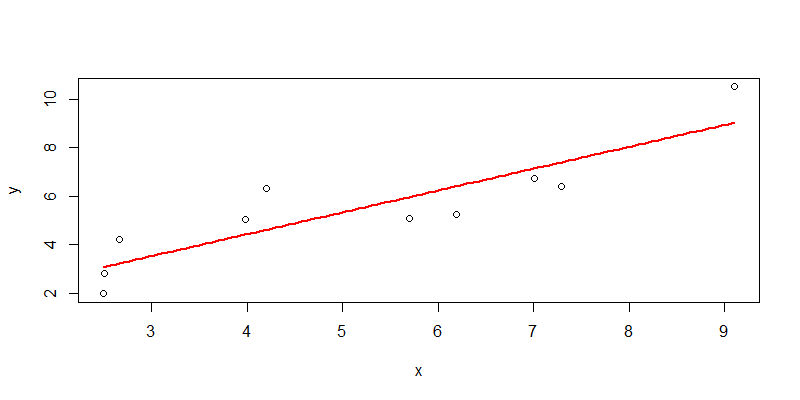## 4. 非线性最小二乘回归

4.1 一元二次函数非线性拟合

``````
# 建立回归模型
> nline1 <- nls(y ~ a*x+b*x^2+c, start = list(a = 1,b = 1,c=2))

# 查看模型结果
> nline1
Nonlinear regression model
model: y ~ a * x + b * x^2 + c
data: parent.frame()
a        b        c
0.008987 0.082188 2.850389
residual sum-of-squares: 9.758

Number of iterations to convergence: 1
Achieved convergence tolerance: 3.024e-06

# 分析模型
> summary(nline1)

Formula: y ~ a * x + b * x^2 + c

Parameters:
Estimate Std. Error t value Pr(>|t|)
a 0.008987   0.977890   0.009    0.993
b 0.082188   0.088760   0.926    0.385
c 2.850389   2.379763   1.198    0.270

Residual standard error: 1.181 on 7 degrees of freedom

Number of iterations to convergence: 1
Achieved convergence tolerance: 3.024e-06
``````

``````
# 模型预测
> npred1<-predict(nline1,newdata=new)

# 可视化
> plot(x,y)
> lines(new\$x,npred1,col="blue",lwd=2)
``````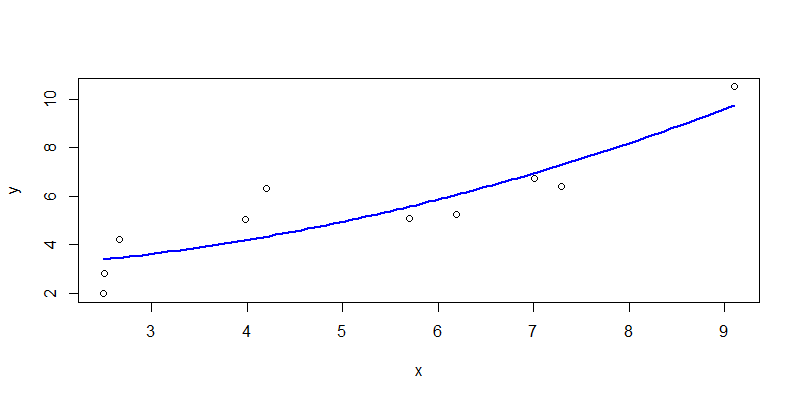4.2 一元三次函数非线性拟合

``````
> nline2 <- nls(y ~ a*x+b*x^2+c*x^3+d, start = list(a = 1,b = 1,c=2,d=1))

# 查看模型结果
> nline2
Nonlinear regression model
model: y ~ a * x + b * x^2 + c * x^3 + d
data: parent.frame()
a       b       c       d
10.011  -1.822   0.110 -12.516
residual sum-of-squares: 3.453

Number of iterations to convergence: 2
Achieved convergence tolerance: 3.983e-08

# 分析模型
> summary(nline2)

Formula: y ~ a * x + b * x^2 + c * x^3 + d

Parameters:
Estimate Std. Error t value Pr(>|t|)
a  10.01051    3.08630   3.244   0.0176 *
b  -1.82152    0.57797  -3.152   0.0198 *
c   0.10998    0.03323   3.310   0.0162 *
d -12.51586    4.88778  -2.561   0.0429 *
---
Signif. codes:  0 ‘***’ 0.001 ‘**’ 0.01 ‘*’ 0.05 ‘.’ 0.1 ‘ ’ 1

Residual standard error: 0.7586 on 6 degrees of freedom

Number of iterations to convergence: 2
Achieved convergence tolerance: 3.983e-08
``````

``````
> npred2<-predict(nline2,newdata=new)
> plot(x,y)
> lines(new\$x,npred2,col="green",lwd=2)
``````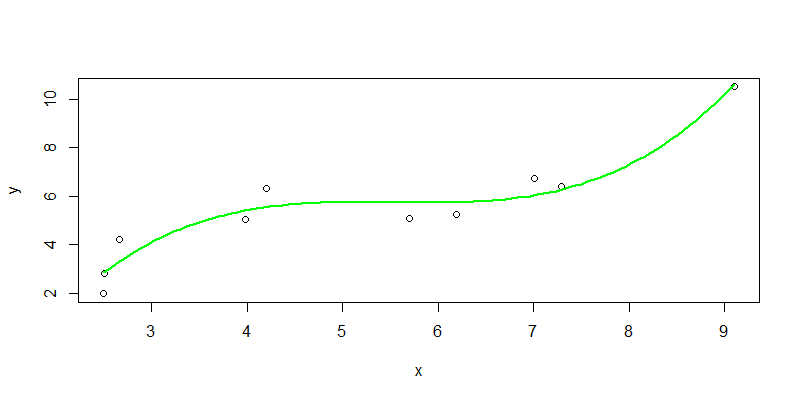4.3 指数函数非线性拟合

``````
> nline3 <- nls(y ~ a*exp(b*x)+c, start = list(a = 1,b = 1,c=2))

> nline3
Nonlinear regression model
model: y ~ a * exp(b * x) + c
data: parent.frame()
a      b      c
0.6720 0.2718 2.2087
residual sum-of-squares: 8.966

Number of iterations to convergence: 17
Achieved convergence tolerance: 7.136e-06

> summary(nline3)

Formula: y ~ a * exp(b * x) + c

Parameters:
Estimate Std. Error t value Pr(>|t|)
a   0.6720     1.2127   0.554    0.597
b   0.2718     0.1764   1.541    0.167
c   2.2087     2.2447   0.984    0.358

Residual standard error: 1.132 on 7 degrees of freedom

Number of iterations to convergence: 17
Achieved convergence tolerance: 7.136e-06
``````

``````
> npred3<-predict(nline3,newdata=new)
> plot(x,y)
> lines(new\$x,npred3,col="yellow",lwd=2)
``````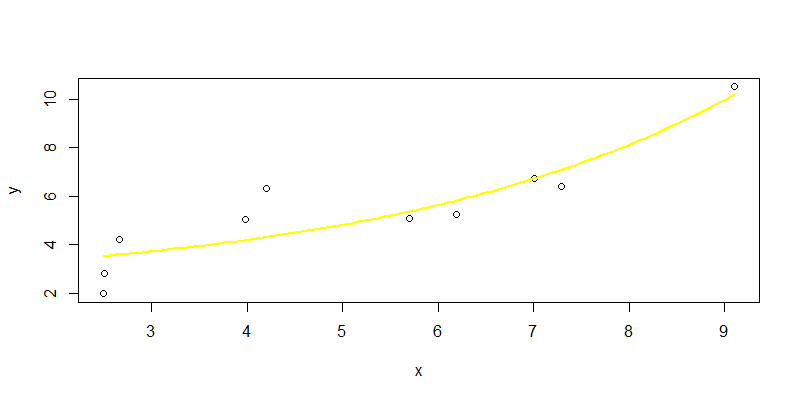4.4 倒数函数非线性拟合

``````> nline4 <- nls(y ~ b/x+c, start = list(b = 1,c=2))

# 查看模型结果
> nline4
Nonlinear regression model
model: y ~ b/x + c
data: parent.frame()
b     c
-17.0   9.5
residual sum-of-squares: 15.74

Number of iterations to convergence: 1
Achieved convergence tolerance: 1.259e-07

> summary(nline4)

Formula: y ~ b/x + c

Parameters:
Estimate Std. Error t value Pr(>|t|)
b  -17.003      4.108  -4.139  0.00326 **
c    9.500      1.077   8.817 2.15e-05 ***
---
Signif. codes:  0 ‘***’ 0.001 ‘**’ 0.01 ‘*’ 0.05 ‘.’ 0.1 ‘ ’ 1

Residual standard error: 1.403 on 8 degrees of freedom

Number of iterations to convergence: 1
Achieved convergence tolerance: 1.259e-07
``````

``````
> npred4<-predict(nline4,newdata=new)
> plot(x,y)
> lines(new\$x,npred4,col="gray",lwd=2)
``````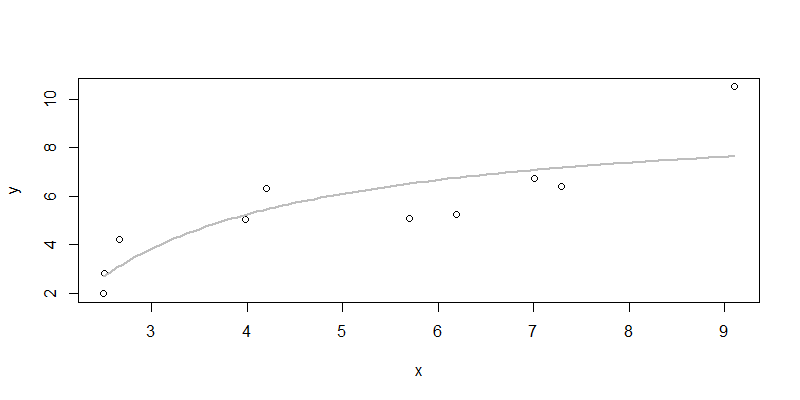4.5 模型优化

``````
> nline5 <- nls(y ~ a*x^3+b*x^5+c*x^7+d*x^9+e*x^11,
+               start = list(a=1,b=2,c=1,d=1,e=1))

# 查看模型结果
> nline5
Nonlinear regression model
model: y ~ a * x^3 + b * x^5 + c * x^7 + d * x^9 + e * x^11
data: parent.frame()
a          b          c          d          e
2.959e-01 -2.064e-02  5.837e-04 -7.324e-06  3.367e-08
residual sum-of-squares: 2.583

Number of iterations to convergence: 3
Achieved convergence tolerance: 2.343e-07

# 模型分析
> summary(nline5)

Formula: y ~ a * x^3 + b * x^5 + c * x^7 + d * x^9 + e * x^11

Parameters:
Estimate Std. Error t value Pr(>|t|)
a  2.959e-01  5.132e-02   5.767   0.0022 **
b -2.064e-02  6.176e-03  -3.341   0.0205 *
c  5.837e-04  2.468e-04   2.365   0.0644 .
d -7.324e-06  3.940e-06  -1.859   0.1222
e  3.367e-08  2.139e-08   1.574   0.1763
---
Signif. codes:  0 ‘***’ 0.001 ‘**’ 0.01 ‘*’ 0.05 ‘.’ 0.1 ‘ ’ 1

Residual standard error: 0.7187 on 5 degrees of freedom

Number of iterations to convergence: 3
Achieved convergence tolerance: 2.343e-07
``````

``````
> npred5<-predict(nline5,newdata=new)
> plot(x,y)
> lines(new\$x,npred5,col="purple3",lwd=2)
``````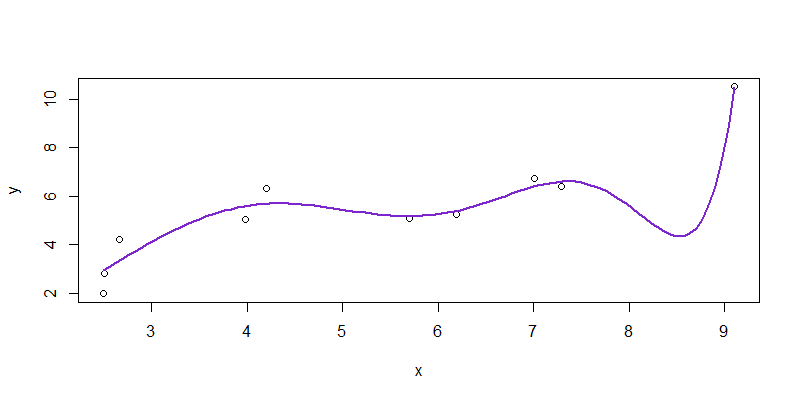4.6 总结

 拟合函数 残差平方和 参数T检验显著性 解释性 颜色 一次函数 10.95334 不显著 好 红色 二次函数 9.758 不显著 好 蓝色 三次函数 3.453 显著 不好 绿色 指数函数 8.966 不显著 不好 黄色 倒数函数 15.74 显著 好 灰色 高次函数 2.583 显著 无法解释 紫色